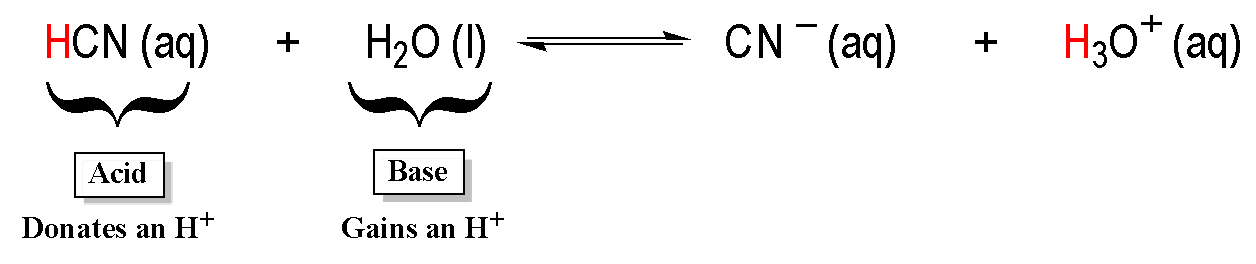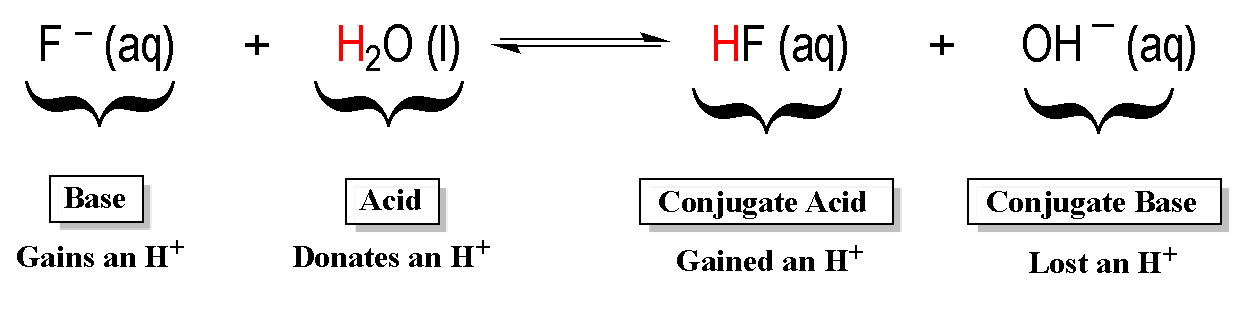Clutch Prep is now a part of Pearson
Ch.15 - Acid and Base EquilibriumWorksheetSee all chapters

# Bronsted Lowry Acid and Base

See all sections
Sections
Identifying Acids and Bases
Arrhenius Acid and Base
Bronsted Lowry Acid and Base
Amphoteric
Lewis Acid and Base
pH and pOH
Ka and Kb
Ionic Salts
Diprotic Acid
Polyprotic Acid

In 1923, Johannes Brønsted and Thomas Lowry developed a new set of definitions for acids and bases.

###### Understanding Bronsted-Lowry Acids and Bases

According to Brønsted and Lowry, an acid was classified as a proton donor while a base was a proton acceptor.

Concept #1: Discussing Brønsted – Lowry Acids and Bases.

Transcript

Hey guys, in this new video, we're going to take a look at another type of acids and bases and probably the most important of the three – Bronsted-Lowry. Here we’re going to say it was in 1923 when Bronsted and Lowry developed a new definition for acids and bases. Arrhenius devised Arrhenius acids and bases near the end of the 1800s. It was in the new century, 1923, where these two guys helped to make that definition a little bit better. We're going to say according to their definition, an acid was considered to be a proton-donor. When we say proton, we mean H+. This first definition still goes in hand with the Arrhenius definition because the Arrhenius acid is something that increases H+ concentration when dissolved in water. Bronsted-Lowry still agreed with that. They said that we agree, an acid should have an H+. Where they disagreed though, Bronsted-Lowry didn't believe that a base needed an OH- to be a base. What they said instead was if the acid donates H+, then the base must accept the H+. Their new definition for a base was bases are proton, which means H+ acceptors. This is where they differed from Arrhenius.
What we're going to say here is we're going to say unlike acids and bases, for Arrhenius, these we could use in solutions that were not just made up of water. We're going to say here, Arrhenius acids say they increase H+. Bronsted-Lowry says give H+. Both agree. You can say that Arrhenius acids are Bronsted-Lowry acids.
We're also going to say here that according to Bronsted-Lowry, a base accepts H+. Why would a base accept H+? Because it has lone pairs or it has a negative charge. Something positive something negative naturally are attracted to one another. This kind of goes in line with Arrhenius a little bit because according to Arrhenius, we have to produce OH-. OH- is negative so it could accept H+. There's a little bit of disagreement but also a little bit of agreement in terms of bases.
Now we’re going to say that Bronsted-Lowry acids and bases are always occurring in pairs, which we call conjugate acid/base pairs. What you need to remember is that conjugate acid/base pairs differ by only one hydrogen. A good example is we have H2O. We could have OH-. We could have H3O+. Here, these two are conjugate acid/base pairs. They’re only different by one H. Water has two H's. OH- only has one. You’re going to say that these two could be conjugate acid/base pairs as well. H3O plus has three H's. H2O has two. They’re only different by one H plus. That's what we mean by conjugate acid/base pairs.Example #1: Write the formula of the conjugate base for the following compound: HSO4-

Transcript

Now, let's take a look at example 1. It says: Write the formula of the conjugate base for the following compound. The following compound is HSO4 negative. Realize when I say conjugate base, I mean remove an H+. Since we're going to be using some of the spaces here guys to write graphs to help us follow along, I'm going to remove myself from the image so we can better focus. Remember, conjugate base means we'll remove H+, so we’ll just do a number line. This number line will help us figure out what the new charge is going to be, because remember, you're not only moving an H. You're moving a positive H. You're causing the charge to change once that H+ comes off. It's important to track and see what your new charge will be. We start off as negative 1. If you're removing an H+, you're removing a positive, you're going to become more negative. Let's remove that H+. Now we're SO4. Because we're going to become a little bit more negative, we fall down to minus 2. SO4 2 negative would be the conjugate base of HSO4 negative.

Example #2: Write the formula of the conjugate acid for the following compound: V2O52-

Transcript

For example 2, we're doing the complete opposite. Now we're looking for a conjugate acid, so that means add an H+. Remember, adding a positive to you makes you more positive, so you become more positive. Number line again.
Let's add an H to it. It becomes HV2O5. Just add the H to the front of the compound. That's all you have to do. Not to the center, to the side. Here, it was negative 2. When I add an H+, it becomes more positive, so it jumps up to negative 1. Our compound now is HV2O5 minus one.
Now that you guys have seen how to do conjugate acids and bases, I want you guys to attempt to do practice question 1 and practice question 2. Good luck!

Practice: Write the formula of the conjugate base for the following compound:

H2Se

Practice: Write the formula of the conjugate for the following compound:

NH2NH2

###### Brønsted – Lowry Reactions

Brønsted – Lowry acid and base reactions create products that are conjugates of the reactants.Example #3: Identify the acid, base, conjugate acid and conjugate base in the following reactions:

HF (aq)  +  H2O (aq)  ⇌ F (aq)  +  H3O+ (aq)

Transcript

Hey guys, in this new video, we're going to put to practice some of the concepts we learned about Bronsted-Lowry acids and bases. Let's take a look at the first example. Here it says: Identify the acid, the base, the conjugate acid and the conjugate base based in the following reactions.
Here we have HF aqueous plus H2O aqueous which gives us F- aqueous plus H3O+. Remember, what does a Bronsted-Lowry acid do? It gives away H+. A Bronsted-Lowry base on the other hand will accept that H+. If we take a look, here we have HF, but then look what happens to the HF. It becomes F-. What must have happened? That HF gave away an H+ to the water. Because it gave its H+ away, it's the acid. The water on the other hand accepted that H+. That's how it became H3O+ over here. Here this must be the base. Then we're going to say HF gives away an H+ to become F-. Since we're taking away an H+, this must be the conjugate base. H2O accepts an H+ to give us H3O+. H3O+ must be the conjugate acid.
We're going to say that these two are connected together as our conjugate acid-base pair. Then these two are connected together as our other set of conjugate acid-base pairs. Remember, we talked about this earlier. They only differ by one hydrogen. If they're different by one H, they’re conjugates of each other.

Example #4: Identify the acid, base, conjugate acid and conjugate base in the following reactions:

CN (aq)  +  H2O (aq)  ⇌  HCN (aq)  +  OH (aq)

Transcript

Now that we've done that one, let’s take a look at example 2. In example 2, we have to do the same thing once again. Here we have CN- but all of a sudden, it becomes HCN. How did that happen? The CN must have accepted an H+. Because it's accepting the H+, it's a base. Who's giving it that H+? It must have been the water. Water gives away an H+ making it an acid. When water gives away the H+, what happens to the water? The water becomes OH-.
Here we would say that this is the conjugate base. Whatever you are, your conjugate is the opposite. If this is a base, this is a conjugate acid. Then based on that, we’d say that these two are conjugates of one another and then these two also. Just realize, water acts as a base in the first example but as an acid in the second. Something that can act as an acid or a base we said was called amphoteric. Water is the best example of an amphoteric species. Depending on what it’s next to, it could act as either an acid or a base. Always be careful. We know that this has to be the base because it's negative. We're still using the rules we've learned before.
If we go back up to water actually, how do we know HF is the acid and H2O is the base? Because remember, they're both going to have H connected to an electronegative element. F and O are both in the same period. Remember, we said when you're in the same period, what do we look at? We look at electronegativity. HF is definitely a stronger acid than H2O. As a result, HF must be the acid. H2O must be the base. The rules we learned earlier play a huge role in what we're doing right now. Here I gave us the product so it’s easy to say who was the acid and who was the base. But on your exam, you may not get that luxury. Your professor might just give you HF plus water, and ask you what are your products. You still have to remember who would be the stronger acid, that person will be the acid. The other one would have to be the base.
Now that we've seen that, let's take a look at practice questions 1 and 2. For this one, we're asking which of the following is a Bronsted-Lowry acid. I'll give you guys a huge help here. Remember, Bronsted-Lowry acid has to have H+ in there. It has to have H. If it doesn't have H, it’s out. Second, for something to give away an H, H needs to be connected to something that is electronegative. If H is connected to an electronegative element, it's going to make a polar bond. If that bond is polar, that means it's reactive. That means that can break it and take that H+ off.
What bonds are not polar? If H was connected to itself, this would be a non-polar bond. As a result, H2 does not represent an acid. Also, who else? We can also say that H, when it's connected to C, their electronegativities are not that far off from one another. Their electronegativities are very similar. We would expect that bond not to be polar. As a result, if you have H connected to carbon, we would expect that to not be an acidic bond. I gave you guys two huge helps to help you figure out which one of these could be a Bronsted-Lowry acid. Use the rules that we've learned earlier to identify something as either an acid or a base. That will help guide you to what’s a Bronsted-Lowry acid for this particular question.

Practice: Which of the following is a Bronsted-Lowry acid?

Practice: Determine the chemical equation that would result when carbonate, CO32-, reacts with water.